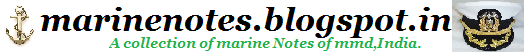Home » , » Principle of Flotation- Archimedes’ Principle,Relationship Between Weight and Buoyancy

# Principle of Flotation- Archimedes’ Principle

“Archimedes” Principle states that when a body is wholly or partially immersed in a fluid it appears to suffer a loss in mass equal to the mass of the fluid it displaces.

### Relative Density

The relationship between weight and volume is called density.  It is defined as ‘mass per unit volume’.  One metric tonne of fresh water has a volume of one cubic metre. Therefore it has a density of 1.000 tonnes/m3. Salt water on the other hand, is heavier.  One cubic metre of salt water weighs 1.025 tonnes, and so salt water has a density of 1.025 tonnes/m3.

The relative density (or specific gravity) of a substance is defined as the ratio of the weight of the substance to the weight of an equal volume of fresh water. In other words, it is simply a comparison of the density of a substance with the density of fresh water.

Density of Substance
R.D.  =    Density of Fresh Water

This is a pure number and has no units.  The R.D. of sea water is therefore 1.025.

# Relationship Between Weight and Buoyancy

Suppose we have a body or block that measures 1 cubic metre and weighs 4000 kg.  If we now lower the block into fresh water, it will displace 1 cubic metre of fresh water - which, as we now know, weighs 1000 kg.  In other words, there is a force acting upwards of 1000 kg and a force acting downwards of 4000 kg: the resultant force has to be 3000 kg downwards.  That is, the block will sink.

If we take the same 4000kg block and mould it into a hollow box with a volume of 5 cubic metres, and then place it in fresh water, it has sufficient volume to displace 5 cubic metres of fresh water.  If the box were now completely submerged, it would experience an upward force of 5000 kg.

However, the downward force of the box is still only 4000 kg, thus the resultant force will be 1000 kg upwards.  In this case the box will rise out of the water to a level where the forces are equal and opposite, that is, with 4 cubic metres under water, and 1 cubic metre still outside water.

Thus for a body to (just) float in water, its weight must be exactly balanced by the force of buoyancy.  If the volume of the body is further increased, it will float with a certain amount outside the water.